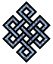#fail2ban bad ip database: ip 58.42.228.170

### | ip database | live view | stats | report | help | api key:

 ip: 58.42.228.170 hostname: 58.42.228.170 country:[CN] China first reported: 21.07.2017 09:14.50 GMT+0200 last reported: 10.12.2018 01:55.05 GMT+0200 time period: 506d 17h 40m 15s total reports: 10 reported by: 4 host(s) filter(s): ssh (8) ssh (2) tor exit node no badips.com db Lookup## port scan of '58.42.228.170':

[-hide]
```# Nmap 6.40 scan initiated Fri Jul 21 09:15:04 2017 as: /usr/bin/nmap -sU -sS -O 58.42.228.170
Nmap scan report for 58.42.228.170
Host is up (0.20s latency).
Not shown: 1000 open|filtered ports, 986 closed ports
PORT      STATE    SERVICE
23/tcp    open     telnet
135/tcp   filtered msrpc
139/tcp   filtered netbios-ssn
443/tcp   open     https
444/tcp   open     snpp
445/tcp   filtered microsoft-ds
1723/tcp  open     pptp
3389/tcp  open     ms-wbt-server
4444/tcp  filtered krb524
5800/tcp  filtered vnc-http
5900/tcp  filtered vnc
8000/tcp  open     http-alt
8081/tcp  open     blackice-icecap
11111/tcp open     vce
No exact OS matches for host (If you know what OS is running on it, see http://nmap.org/submit/ ).
TCP/IP fingerprint:
OS:SCAN(V=6.40%E=4%D=7/21%OT=23%CT=1%CU=%PV=N%G=Y%TM=5971ABA7%P=x86_64-pc-l
OS:inux-gnu)SEQ(SP=108%GCD=1%ISR=105%TI=I%CI=I%TS=U)SEQ(SP=108%GCD=1%ISR=10
OS:5%TI=I%TS=U)OPS(O1=M5B4%O2=M400%O3=M218%O4=M200%O5=M218%O6=M100)WIN(W1=2
OS:000%W2=2000%W3=2000%W4=2000%W5=2000%W6=2000)ECN(R=Y%DF=N%TG=FF%W=2000%O=
OS:M5B4%CC=N%Q=)T1(R=Y%DF=N%TG=FF%S=O%A=S+%F=AS%RD=0%Q=)T2(R=N)T3(R=Y%DF=N%
OS:TG=FF%W=1FC4%S=O%A=O%F=A%O=%RD=0%Q=)T4(R=Y%DF=N%TG=FF%W=2000%S=A%A=Z%F=R
OS:%O=%RD=0%Q=)T5(R=Y%DF=N%TG=FF%W=0%S=Z%A=S+%F=AR%O=%RD=0%Q=)T6(R=Y%DF=N%T
OS:G=FF%W=0%S=A%A=Z%F=R%O=%RD=0%Q=)T7(R=Y%DF=N%TG=FF%W=0%S=Z%A=S%F=AR%O=%RD
OS:=0%Q=)U1(R=N)IE(R=N)

OS detection performed. Please report any incorrect results at http://nmap.org/submit/ .
# Nmap done at Fri Jul 21 09:22:15 2017 -- 1 IP address (1 host up) scanned in 432.60 seconds
```
```Σ = 87 | Δt = 0.0048420429229736s
```